1. /
2. CBSE
3. /
4. Class 05
5. /
6. Mathematics
7. /
8. NCERT Solutions for Class...

# NCERT Solutions for Class 5 Maths Be My Multiple### myCBSEguide App

Download the app to get CBSE Sample Papers 2023-24, NCERT Solutions (Revised), Most Important Questions, Previous Year Question Bank, Mock Tests, and Detailed Notes.

NCERT solutions for Class 5 Mathematics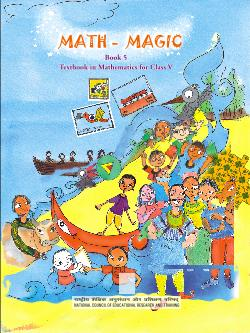## NCERT Solutions for Class 5 Maths Be My Multiple

###### 1. The hungry cat is trying to catch Kunjan the mouse. Kunjan is now on the 14th step and it can jump 2 steps at a time. The cat is on the third step. She can jump 3 steps at a time. If the mouse reaches 28 it can hide in the hole. Find out whether the mouse can get away safely!

(a) The steps on which the mouse jumps.

(b) The steps on which the cat jumps.

(c) The steps on which both the cat and the mouse jump.

(d) Can the mouse get away?

Ans. (a)The steps on which the mouse jumps are 16, 18, 20, 22, 24, 26 and 28.

(b) The steps on which the cat jumps are 3,6,9, 12, 15, 18, 21, 24, 27.

(c) The steps on which both the cat and the mouse jump are 18 and 24.

(d) The mouse can get away safely as they do not come together at the steps 18 and 24.

###### 2. If the cat starts from the 5th step and jumps five steps at a time and the mouse starts from the 8th step and jumps four steps at a time, can the mouse get away?

Ans. In this case:

(a) The steps on which the mouse jumps are 8, 12, 16, 20, 24 and 28

(b) The steps on which the cat jumps are 5, 10, 15, 20 and 25.

(c) The step on which both of the cat and mouse jump is 20.

(d) Since their 4th jump is on step 20 together so the mouse will not get away safely.

NCERT Solutions for Class 5 Maths Be My Multiple

###### 3. Who is Monto waiting for?

Monto cat is waiting for somebody. Do you know for whom he is waiting? There is a   trick to find out.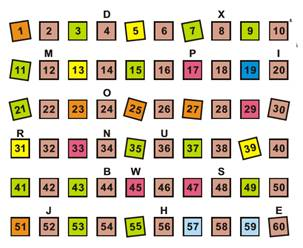###### Mark with a red dot all the numbers which can be divided by 2. Mark a yellow dot on the numbers which can be divided by 3 and a blue dot on the numbers which can be divided by 4. Which are the boxes which have dots of all three colours? What are the letters on top of those boxes? Write those letters below in order.

Ans. Let us mark the coloured dots are desired on the above number:

Red dot will appear on the numbers: 2, 4, 6,8, 10, 12, 14, 16, 18, 20, 22, 24, 26, 28, 30, 32,      34, 36, 38, 40, 42, 44, 46,48, 50, 52, 54, 56, 58 and 60.

Yellow dot will appear on the numbers: 3,6, 9, 12, 15, 18, 21, 24, 27, 30,33, 36, 39, 42, 45, 48, 51, 54, 57 and 60.

Blue dot will appear on the numbers: 4, 8, 12, 16, 20, 24, 28, 32, 36, 40, 44, 48, 52, 56 and 60.

All the three coloured dots will appear on the boxes: 122, 24, 36, 48 and 60.

The respective letters on the top of these boxes are: M, O, U, S and E.

Writing these letters in order: MOUSE.

###### 4. To play this game, everyone stands in a circle. One player calls out ‘one’. The next player says ‘two’ and so on. A player who has to call out 3 or a number which can be divided by 3 has to say ‘Meow’ instead of the number. One who forgets to say ‘Meow’ is out of the game. The last player left is the winner.

(a) Which numbers did you replace with ‘Meow’?

Ans. The number which will be replaced with ‘Meow’ are:

3,6, 9, 12, 15, 18, 21, 24, 27, 30…

(b) Now, which numbers did you replace with ‘Meow’?

Ans. The numbers which will be replaced with ‘Meow’ are:

4, 8, 12, 16, 20, 24, 28, 32…

(c) Write any ten multiple of 5.

Ans. Ten multiple of 5 are: 5, 10, 15, 220, 25, 30, 35, 40, 45 and 50.

NCERT Solutions for Class 5 Maths Be My Multiple

###### 5. Tamarind seeds Sunita took some tamarind (imli) seeds. She made groups of five with them, and found that one seed was left over. She tried making groups of six and groups of four. Each time one seed was left over. What is the smallest number of seeds that Sunita had?

Ans. We know that the smallest number divisible by 5,6 and 4 is their smallest common multiple. Therefore, the required number be 1 more than this smaller common multiple.

The smallest common multiple of 5,6 and 4 =60

Therefore, the required number =60+1=61

Hence, the smallest number of seeds Sunita had 61.

NCERT Solutions for Class 5 Maths Be My Multiple

###### 6. There are 18 bangles on the rod. Meena trying to group them. She can put them in groups of 2, 3,6, 9 and 18 – without any bangle being left.

(a) How many bangles will she have if she makes groups of 1 bangle each?

Now, complete the table, for different numbers of bangles. For each number see what different groups can be made.

Ans. Meena will have 18 groups of 1 bangle each. The complete table is on the given here:

 Number of bangles Different groups we can make 18 1,2,3, 6, 9, 18 24 1, 2, 3, 4, 6, 8, 12, 24 5 1,5 9 1,3,6, 9 7 1,7 2 1,2 10 1,2,5,10 1 1,1 20 1,2,4,5,10,20 13 1,13 21 1,3,7,21

NCERT Solutions for Class 5 Maths Be My Multiple

###### 7.  Complete the multiplication chart given here: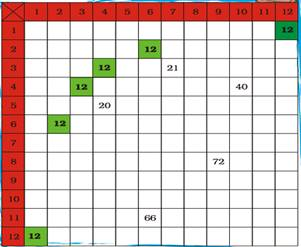Ans.

 1 2 3 4 5 6 7 8 9 10 11 12 1 1 2 3 4 5 6 7 8 9 10 11 12 2 2 4 6 8 10 12 14 16 18 20 22 24 3 3 6 9 12 15 18 21 24 27 30 33 36 4 4 8 12 16 20 24 28 32 36 40 44 48 5 5 10 15 20 25 30 35 40 45 50 55 60 6 6 12 18 24 30 36 42 48 54 60 66 72 7 7 14 21 28 35 42 49 56 63 70 77 84 8 8 16 24 32 40 48 56 64 72 80 88 96 9 9 18 27 36 45 54 63 72 81 90 99 108 10 10 20 30 40 50 60 70 80 90 100 110 120 11 11 22 33 44 55 66 77 88 99 110 121 132 12 12 24 36 48 60 72 84 96 108 120 132 144

NCERT Solutions for Class 5 Maths Be My Multiple

###### 8. Look at the green boxes in the chart. These show how we can get 12 by multiplying different numbers. 12 = 4 × 3, so 12 is a multiple of both 4 and 3. 12 is also a multiple of 6 and 2, as well as 12 and 1. We say 1, 2, 3, 4, 6, 12 are factors of 12.

(a) What are the factors of 10?

Can you do this from the chart?

(b) What are the factors of 36?

(c) Find out all the factors of 36 from the multiplication chart.

(d) What is the biggest number for which you can find the factors from this chart?

(e)  What can you do for number bigger than chart?

Ans.(a) The factors of 10 are 1, 2, 5, 10.

Yes, we can find these factors from the above chart.

(b) The factors of 36 are 1, 2,3,4, 6, 9, 12, 18, 36.

(c) From the multiplication chart, we find that

36=3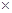12, 36=49, 36=66, 36=94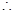3,4,6, 9, 12 are factors of 36 as found from the above chart.

(d) The biggest number for which we can find the factors from this chart is 144.

(e) We can find the factors of bigger number just by reducing to smaller numbers by division and then using and then using the above chart.

NCERT Solutions for Class 5 Maths Be My Multiple

###### 9. There is a garden in Anu’s house. In the middle of the garden there is a path. They decided to tile the path using tiles of length 2 feet, 3 feet and 5 feet. The mason tiled the first row with 2 feet tiles, the second row with 3 feet tiles and the third row with 5 feet tiles. The mason has not cut any of the tiles. Then what is the shortest length of the path?

Ans. The shortest length of the path is the smallest common multiple of 2,3 and 5.

The shortest length of the path=30 feet.

NCERT Solutions for Class 5 Maths Be My Multiple

###### 10.  Manoj has made a new house. He wants to lay tiles on the floor. The size of the room is 9 feet12 feet. In the market, there are three kinds of square tiles: 1 foot × 1 foot, 2 feet2 feet and 3 feet3 feet. Which size of tile should he buy for his room, so that he can lay it without cutting?

Ans. Since, the tile of size 2feet2 feet cannot be laid down on the width side of the room’s without cutting so this type of the tile should not be purchased. He can buy tiles of size 1foot1 foot or 3 feet3 feet as these can be laid down without cutting on the floor.

NCERT Solutions for Class 5 Maths Be My Multiple

###### 11. Rani, Geetha and Naseema live near each other. The distance from their houses to the road is 90 feet. They decided to tile the path to the road. They all bought tiles of different designs and length. Rani bought the shortest tile, Geetha bought the middle sized one and Naseema bought the longest one. If they could tile the path without cutting any of the tiles, what is the size of the tiles each has bought? Suggest 3 different solutions. Explain how you get this answer.

Ans. Let us write down the factors of 90

90=190, 90=245, 90=330

90=518, 90=615, 90=910

Since, we have to lay down the tiles on the path of 90 feet without cutting so any of the size indicated by the factors will do.

Therefore, the possible solution to tile the path using tiles of length.

1foot, 2 feet, 3 feet; 2 feet, 3 feet, 5 feet; 3feet, 5 feet, 6 feet etc.

## NCERT Solutions for Class 5 Mathematics Chapter 6 Be My Multiple

NCERT Solutions Class 5 Maths PDF (Download) Free from myCBSEguide app and myCBSEguide website. Ncert solution class 5 Maths includes text book solutions from Class 5 Maths Book . NCERT Solutions for CBSE Class 5 Maths have total 14 chapters. 5 Maths NCERT Solutions in PDF for free Download on our website. Ncert Maths class 5 solutions PDF and Maths ncert class 5 PDF solutions with latest modifications and as per the latest CBSE syllabus are only available in myCBSEguide.

## CBSE app for Students

To download NCERT Solutions for Class 5 Maths, Social Science Computer Science, Home Science, Hindi English, Maths Science do check myCBSEguide app or website. myCBSEguide provides sample papers with solution, test papers for chapter-wise practice, NCERT solutions, NCERT Exemplar solutions, quick revision notes for ready reference, CBSE guess papers and CBSE important question papers. Sample Paper all are made available through the best app for CBSE students and myCBSEguide website.Test Generator

Create question paper PDF and online tests with your own name & logo in minutes.myCBSEguide

Question Bank, Mock Tests, Exam Papers, NCERT Solutions, Sample Papers, Notes